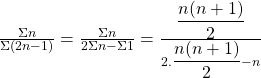# TS Polycet (Polytechnic) 2015 Previous Question Paper with Answers And Model Papers With Complete AnalysisTS Polycet (Polytechnic) 2015 Previous Question Paper with Answers And Model Papers With Complete Analysis
TS Polycet (Polytechnic) Previous Year Question Papers And Model Papers:
While preparing for TS Polycet (Polytechnic), candidates must also refer to the previous year question papers of the same. Scoring well in TS Polycet (Polytechnic) and understanding weaknesses and strengths in the respective sections.

TS Polycet (Polytechnic) Previous Year Question Papers can be found on this page in PDF format. Students taking the exam to get into some of the best Polytechnic colleges/institutes in the state of Andhra Pradesh may practice these papers to get a clear idea of the structure of the exam, marking scheme, important topics, etc.

## Section — I MATHEMATICS

Q). The sum of two numbers is 1000 and the difference between their squares is 256000. Find the numbers.
A) 630,370
B) 628,372
C) 626,374
D) 620,380

B) 628,372
Explanation: Let the two numbers are x, y.
Given that x + y = 1000 ……….(1)
x2 — y2 = 256000
(x—y) (x + y)= 256000 (x-y) (1000) = 256000
x-y= 256 ……………………(2)
Solve(1)&(2)
x + y = 1000
x – y = 256
2x = 1256
⇒ x = 628
(1) ⇒ 628 + y = 1000
∴ y = 1000 – 628 ⇒ y = 372
∴ x = 628, y = 372

Q). Solve : 141x + 93y = 189, 93x+ 141y=45
A) (0,7)
B) (1,-1)
C) (1,2)
D) (2,-1)

D) (2,-1)
Explanation: 141x + 93y=189 ………………….(1)
93x + 141y = 45 …………………(2)
Solve (1), (2)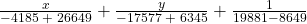x/22464 = y/-11232 = 1/11232
∴x = 2, y = – 1
∴ (x, y) = (2, – 1)

Q). If the system of equations 2x + 3y = 7, 2ax + (a + b) y = 28 has infinitely many solutions, then
A) a = 2b
B) b = 2a
C) a + 2b = 0
D) 2a + b = 0

B) b = 2a
Explanation: a1/a2 = b1/b2 = c1/c2
⇒2/2a = 3/a+b = -7/-28
⇒1/a = 3/a+b = 1/4
⇒1/a = 1/4 ⇒a=4
∴3/a+b = 1/4⇒a+b =12
⇒4+b =12
⇒B = 8
∴b = 8 = 2(4) = 2a
∴b = 2a

Q). The squares of two consecutive integers differ by 13, then the largest integer is
A) 12
B) 6
C) 7
D) 13

C) 7
Explanation: Let the two consecutive integers are x, x + 1.
Given that
(X+1)2 = x2 = 13
x2+ 1 +2x- x2= 13
1 + 2x = 13
2x = 12 ⇒ x = 6
∴ Largest integer = x+ 1 = 6+ 1 = 7.

Q). If 2x -3/x = 5, then x =
A) 1/2 ,3
B) -1/2, -3
C) -1/2, 3
D) 1/2, -3

C) -1/2, 3
Explanation: 2x- 3/x = 5
2 x2-3/x = 5
2 x2 – 3 = 5x
⇒ 2 x2 – 5x -3 = 0 ⇒ 2 x2-6x + x- 3 = 0
⇒ 2x(x-3) + 1(x-3) = 0 ⇒ (2x + 1) (x-3) = 0
∴ x = 3 or x = -1/2

Q). If a x2 + bx + c is a perfect square, then b2=
A) 4ac
B) ac
C) 2ac
D) √2ac

A) 4ac
Explanation: a x2 + bx + c is a perfect square then b2 = 4ac

Q). If nth terms of the progressions 63, 65,67,……………….. and 3, 10, 17,……………….. are same, then n =
A) 10
B) 11
C) 12
D) 13

D) 13
Explanation: I progression.: nth term an = a + (n – 1) d
= 63+(n-1)2
= 63 + 2n — 2 = 2n + 61
II progression: nth term an = a + (n – 1) d = 3 + (n – 1) 7
= 3 + 7n-7 = 7n – 4
Given that 2n + 61 = 7n – 4
7n-2n = 61 +4
5n = 65 ⇒ n = 13

Q). If a, b and c are in AP and a > 0, then…………………..are in GP.
A) aa, bb, cc
B) ac, ba, cb
C) ab, bc, ca
D) aa, ab, ac

D) aa, ab, ac
Explanation: a, b, c are in AP ⇒ 2b = a + c
a2b = aa+c
(ab)2 = aa .ac
aa, ab, ac are in G.P.

Q). In a GP, third-term is 24 and sixth-term is 192, then tenth-term is
A) 3072
B) 2456
C) 1346
D) 3126

A) 3072
Explanation: t3 = 24 ⇒ ar2 = 24 …………………….(1)
t6 = 192 ⇒ ar5 = 192 ………………………. (2)
(2)/(1) ⇒ ar5/ar2 = 192/24
⇒ r3 = 8⇒ r = 2
(1) ⇒ a(2)2 = 24 ⇒ 4a = 24 ⇒ a = 6
t10 = ar9 = 6(2)9 = 3072

Q).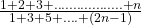A)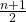B)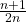C) n(n+1)
D) None

B)Explanation:=